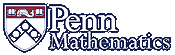Math 320### Introduction

This course focuses on finding numerical solutions to mathematics problems for which there are no "simple" solutions. Ultimately, one uses a computer program, which either you write yourself (in some computer language) or from a commercial package such as Maple, Mathematica, and Matlab. To get numerical results you trust, you can't just press a button. You need a fundamental understanding of the,mathematical issues of what can go wrong ("an expert is someone who has made all of the mistakes"). That is what this course is all about.

There are four fundamental complications:

1. On a computer you only have a finite number of decimal places. The basic statement "a=b" (using absolute error) only means that |a-b| is "small", where the interpretation of "small" depends on the computer hardware you are using. [It is often wiser to require that the relative error |a-b|/|a| be small).
One wrinkle here is that the input numerical data for a problem are themselves usuallly approximations. Thus one needs to understand how much small changes in the data will change the "solution" of a problem.

2. Even if in principle there is an exact solution, perhaps an integer, the problem may be so large that it cannot be solved by the largest computer in hundreds of years.
One notable example is finding the prime factors in an integer with 100 digits. This arises in modern cryptography, where a basic open question is either to find a speedy factorization algorithm or prove that no such algorithm exists. [If you can answer this, your work immediately becomes immortal.]

Even if there is, in principle, an exact solution, finding speedy accurate algorithms is a basic task. An example is solving a system of 10,000 linear algebraic equations with 10,000 unknowns where the coefficients are integers and you already know that there is a unique solution.

3. For many standard problems, such as computing 1/7 as a decimal, solving x2 = 2, finding the (possibly complex) roots of a polynomial, evaluating a definite integral (such as in computing the area of a circle), or solving a differential equation, a limiting process is involved so good approximation techniques are the most one can hope.

4. While a computer may be fast and have lots of memory, it is not infinite. It is easy to naively exhaust your computer's resources. A simple example is to compute the sum
SN := 1 + 1/2 + 1/3 + 1/4 + ... + 1/N
to find a number N so that SN > 100. You should live so long.
General Comments The creative side of science is an emphasis on discovery, reasoning, and effective communication: convincing others.

This course is about thinking, attempting to understand. It is for students who enjoy thinking hard, even when completely stumped. Then understanding (when it comes) is all the sweeter.

Intuition and computational skill will be essential in the discovery and presentation of your ideas.

The concept of a proof will be vital. There is nothing exotic about a proof. It is simply convincing someone else about your reasoning. You give different proofs to different people, depending on their background — and how well you know them. The first and most important person to convince is yourself.

Our small class size permits informal discussion, Sometimes the entire class will work together on a given problem. Homework is intended to be thought-provoking.

To be successful in this course, you should be present for all class meetings and plan to take good notes.

Recommended Text: Numerical Analysis, 10th Edition,   by Richard L. Burden, J. Douglas Faires, and Annette M. Burden,   ISBN-13: 978-1305253667
You can of course buy this online. Moreover, I noticed PDF versions of the 9th Edition online. It will be entirely adequate (in fact, I have not yet even seen the 10th edition).
One reason I chose this text is because it contains a number of useful computer examples that we will not have time to cover in class.
Note that our course will be at a somewhat higher mathematical level than this test.

Computer Programs: I will assume you already have experience with some computer software. If not, most students can fairly quickly learn enough to manage. Ask your friends. Some possibilities are lower level programs such as C, perl, ..., as well as higher level packages like Maple, Mathematica, and Matlab,. Student versions of many packages are available to Penn students

NOTE: Maple is available on all our public labs. Also, SAS undergraduates have access to MATLAB (https://www.sas.upenn.edu/computing/matlab-student) and Mathematica (https://www.sas.upenn.edu/computing/mathematica-student) for non-research academic work. Those pages will guide them through the license request and installation process. Wharton and SEAS undergraduates need to acquire licenses through their respective schools, but we include links to the instructions on both pages.